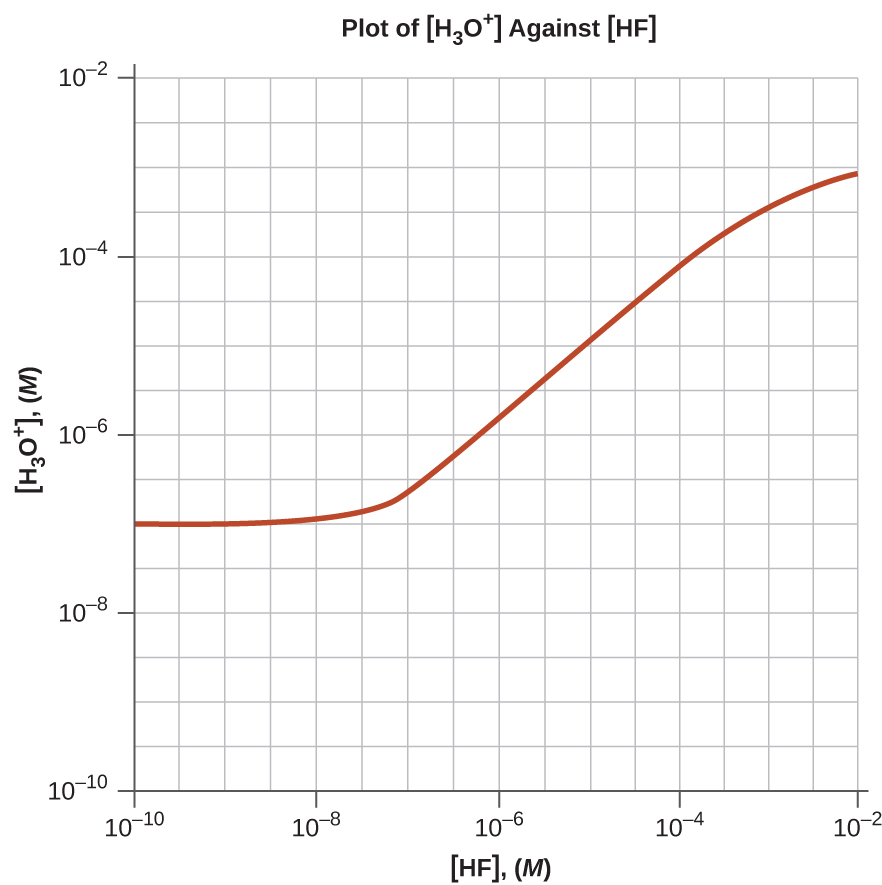# ACID–BASE TITRATIONS ASSIGNMENTS

1. Explain how to choose the appropriate acid-base indicator for the titration of a weak base with a strong acid.
2. Explain why an acid-base indicator changes color over a range of pH values rather than at a specific pH.
3. Why can we ignore the contribution of water to the concentrations of H3O+ in the solutions of following acids:

0.0092 M HClO, a weak acid

0.0810 M HCN, a weak acid

0.120 M$\text{Fe(H}_2\text{O})_6^{\;\;2+}$ a weak acid, Ka = 1.6 × 10−7

but not the contribution of water to the concentration of OH?

4. We can ignore the contribution of water to the concentration of OH in a solution of the following bases:

0.0784 M C6H5NH2, a weak base

0.11 M (CH3)3N, a weak base

but not the contribution of water to the concentration of H3O+?

5. Draw a curve for a series of solutions of HF. Plot [H3O+]total on the vertical axis and the total concentration of HF (the sum of the concentrations of both the ionized and nonionized HF molecules) on the horizontal axis. Let the total concentration of HF vary from 1 × 10−10M to 1 × 10−2M.
6. Draw a curve for a series of solutions of NH3. Plot [OH] on the vertical axis and the total concentration of NH3 (both ionized and nonionized NH3 molecules) on the horizontal axis. Let the total concentration of NH3 vary from 1 × 10−10M to 1 × 10−2M.
7. Calculate the pH at the following points in a titration of 40 mL (0.040 L) of 0.100 M barbituric acid (Ka = 9.8 × 10−5) with 0.100 M KOH.

(a) no KOH added

(b) 20 mL of KOH solution added

(c) 39 mL of KOH solution added

(d) 40 mL of KOH solution added

(e) 41 mL of KOH solution added

8. The indicator dinitrophenol is an acid with a Ka of 1.1 × 10−4. In a 1.0 × 10−4Msolution, it is colorless in acid and yellow in base. Calculate the pH range over which it goes from 10% ionized (colorless) to 90% ionized (yellow).

SOLUTIONS

1. At the equivalence point in the titration of a weak base with a strong acid, the resulting solution is slightly acidic due to the presence of the conjugate acid. Thus, pick an indicator that changes color in the acidic range and brackets the pH at the equivalence point. Methyl orange is a good example.

3. In an acid solution, the only source of OH ions is water. We use Kw to calculate the concentration. If the contribution from water was neglected, the concentration of OHwould be zero.

5.7. (a) pH = 2.50;

(b) pH = 4.01;

(c) pH = 5.60;

(d) pH = 8.35;

(e) pH = 11.08